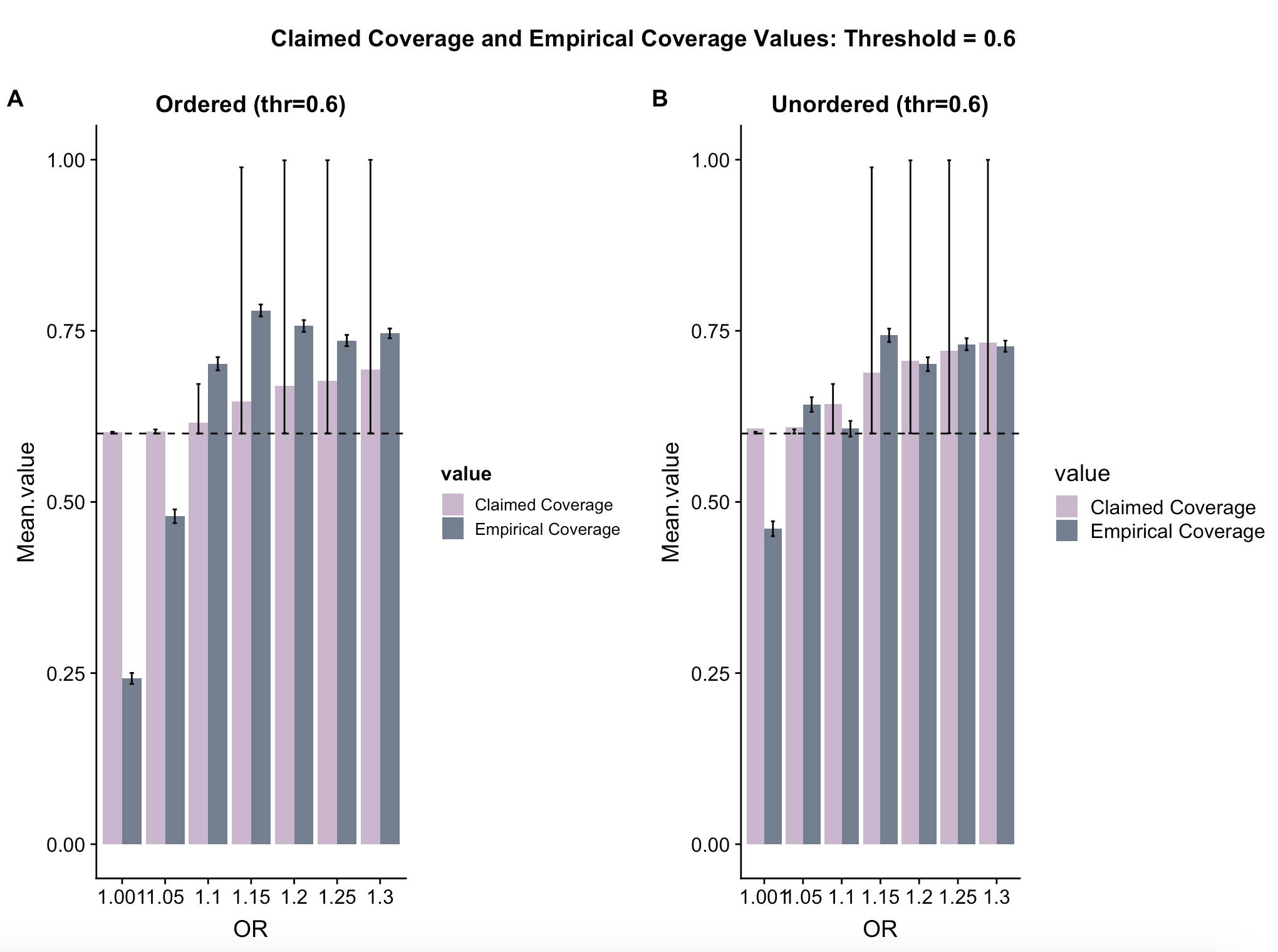EMPIRICAL COVERAGE: Average coverage from simulations (“true coverage”)

CLAIMED COVERAGE: Size of the credible set

CORRECTED COVERAGE: GAM predicted coverage

#### Empirical and Claimed Coverage Plots From Simulations Using Real-World Data

• A simulation dataset was obtained using real world minor allele frequency and linkage disequilibrium data from the UK10K project.

• The data was split into ordered and unordered datasets, with 300,000 simulations in each.

(Wrong) Simulation method:

1. Obtain freq, a reference haplotype matrix based on real world MAF and LD data.

2. For a fixed OR (odds ratio) and N (sample size), generate nrep (=100) posterior probability systems.
3. For each of these, form two credible sets: one using ordered posterior probabilities and one using unordered posterior probabilities. Obtain the size (claimed coverage) and whether the CV is in the set for each credible set (covered). The same CV is chosen as causal for these systems.
4. In the form a table, report: order (whether the posterior probabilities were ordered), threshold, size, nvar (number of variants in the credible set), covered (whether the CV was in the credible set), N (sample size) and OR.
5. Replicate steps 2-4 many times for varying thresholds (0.6, 0.8, 0.9, 0.95) ORs (1.001, 1.05, 1.1, 1.15, 1.2, 1.25, 1.3) and sample sizes (N0=N1=2000, 5000, 10000, 50000).

• The bar charts below show the mean claimed coverage and the empirical coverage (proportion of simulations where the CV was in the set) for each odds ratio and threshold value for ordered and unordered methods. The dashed line shows the threshold in each plot.

• The error bars for coverage show the Jeffreys credible interval. This uses the Jeffreys prior (non-informative and invariant under transformation) to obtain a 95% credible interval for the posterior probabilties. Here, \[Prior: Beta(1/2,1/2),\] \[Posterior: Beta(x+1/2,n-x+1/2)\] where \(x\) is the number of successes (covered=1) and \(n\) is the number of trials (nrow(dataset)).

• The error bars for size are the 5’th and 95’th percentiles. They are asymmetric as our data is asymmetric, the value for size cannot extend beyond 1.